SNVSA99C July   2015  – November 2017

PRODUCTION DATA.

1. Features
2. Applications
3. Description
4. Revision History
5. Pin Configuration and Functions
6. Specifications
7. Detailed Description
1. 7.1 Overview
2. 7.2 Functional Block Diagram
3. 7.3 Feature Description
4. 7.4 Device Functional Modes
8. Applications and Implementation
1. 8.1 Application Information
2. 8.2 Typical Applications
1. 8.2.1 Design Requirements
2. 8.2.2 Detailed Design Procedure
3. 8.2.3 Application Performance Curves
9. Power Supply Recommendations
10. 10Layout
1. 10.1 Layout Guidelines
2. 10.2 Layout Example
11. 11Device and Documentation Support
12. 12Mechanical, Packaging, and Orderable Information

• PWP|16
• PWP|16

## 8 Applications and Implementation

NOTE

Information in the following applications sections is not part of the TI component specification, and TI does not warrant its accuracy or completeness. TI’s customers are responsible for determining suitability of components for their purposes. Customers should validate and test their design implementation to confirm system functionality.

### 8.1 Application Information

The LM43600-Q1 is a step down DC-to-DC regulator. It is typically used to convert a higher DC voltage to a lower DC voltage with a maximum output current of 0.5 A. The following design procedure can be used to select components for the LM43600-Q1. Alternately, the WEBENCH® software may be used to generate complete designs. When generating a design, the WEBENCH® software utilizes iterative design procedure and accesses comprehensive databases of components. See for more details.

### 8.2 Typical Applications

The LM43600-Q1 only requires a few external components to convert from a wide range of supply voltage to output voltage. Figure 44 shows a basic schematic when BIAS is connected to VOUT . This is recommended for VOUT ≥ 3.3 V. For VOUT < 3.3 V, BIAS should be connected to ground, as shown in Figure 45.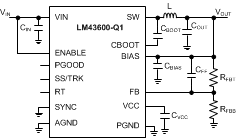Figure 44. LM43600-Q1 Basic Schematic for
VOUT ≥ 3.3 V, Tie BIAS to VOUT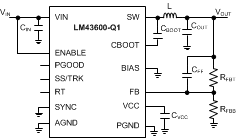Figure 45. LM43600-Q1 Basic Schematic for
VOUT < 3.3 V, Tie BIAS to Ground

The LM43600-Q1 also integrates a full list of optional features to aid system design requirements, such as precision enable, VCC UVLO, programmable soft start, output voltage tracking, programmable switching frequency, clock synchronization and power-good indication. Each application can select the features for a more comprehensive design. A schematic with all features utilized is shown in Figure 46.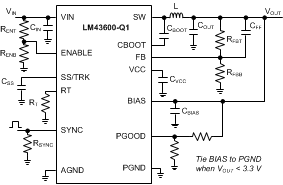Figure 46. LM43600-Q1 Schematic with All Features

The external components have to fulfill the needs of the application, but also the stability criteria of the device's control loop. The LM43600-Q1 is optimized to work within a range of external components. The LC output filter's inductance and capacitance have to be considered in conjunction, creating a double pole, responsible for the corner frequency of the converter. Table 2 can be used to simplify the output filter component selection.

### Table 2. L, COUT and CFF Typical Values

FS (kHz) L (µH)(1) COUT (µF) (2) CFF (pF) (3)(4) RT (kΩ) RFBB (kΩ) (3)(4)
VOUT = 1 V
200 22 500 none 200 100
500 10 330 none 80.6 or open 100
1000 4.8 180 none 39.2 100
2200 2.2 100 none 17.8 100
VOUT = 3.3 V
200 68 220 44 200 442
500 22 100 33 80.6 or open 442
1000 15 47 18 39.2 442
2200 6.8 27 12 17.8 442
VOUT = 5 V
200 82 150 68 200 255
500 33 66 33 80.6 or open 255
1000 18 33 22 39.2 255
2200 6.8 22 18 17.8 255
VOUT = 12 V
200 150 33 see note (5) 200 90.9
500 56 22 47 80.6 or open 90.9
1000 27 15 33 39.2 90.9
Inductor values are calculated based on typical VIN = 12 V. For VOUT = 12 V, typical VIN = 24 V.
All the COUT values are after derating. Add more when using ceramics.
RFBT = 0 Ω for VOUT = 1 V. RFBT = 1 MΩ for all other VOUT settings.
For designs with RFBT other than 1 MΩ, adjust CFF such that (CFF × RFBT) is unchanged and adjust RFBB such that (RFBT / RFBB) is unchanged.
High ESR COUT gives enough phase boost and CFF not needed.

#### 8.2.1 Design Requirements

A detailed design procedure is described based on a design example. For this design example, use the parameters listed in Table 3 as the input parameters.

### Table 3. Design Example Parameters

DESIGN PARAMETER VALUE
Input voltage VIN 12 V typical, range from 3.8 V to 36 V
Output voltage VOUT 3.3 V
Input ripple voltage 400 mV
Output ripple voltage 30 mV
Output current rating 0.5 A
Operating frequency 500 kHz
Soft-start time 10 ms

#### 8.2.2.1 Custom Design With WEBENCH® Tools

Click here to create a custom design using the LM43600-Q1 device with the WEBENCH® Power Designer.

1. Start by entering the input voltage (VIN), output voltage (VOUT), and output current (IOUT) requirements.
2. Optimize the design for key parameters such as efficiency, footprint, and cost using the optimizer dial.
3. Compare the generated design with other possible solutions from Texas Instruments.

The WEBENCH Power Designer provides a customized schematic along with a list of materials with real-time pricing and component availability.

In most cases, these actions are available:

• Run electrical simulations to see important waveforms and circuit performance
• Run thermal simulations to understand board thermal performance
• Export customized schematic and layout into popular CAD formats
• Print PDF reports for the design, and share the design with colleagues

#### 8.2.2.2 Output Voltage Setpoint

The output voltage of the LM43600-Q1 device is externally adjustable using a resistor divider network. The divider network is comprised of top feedback resistor RFBT and bottom feedback resistor RFBB. The following equation is used to determine the output voltage of the converter:

Equation 11.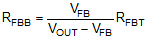Choose the value of the RFBT to be 1 MΩ to minimize quiescent current to improve light load efficiency in this application. With the desired output voltage set to be 3.3 V and the VFB = 1.016 V, the RFBB value can then be calculated using Equation 11. The formula yields a value of 444.83 kΩ. Choose the closest available value of 442 kΩ for the RFBB. See Adjustable Output Voltage for more details.

#### 8.2.2.3 Switching Frequency

The default switching frequency of the LM43600-Q1 device is set at 500 kHz when RT pin is open circuit. The switching frequency is selected to be 500 kHz in this application for one less passive components. If other frequency is desired, use Equation 12 to calculate the required value for RT.

Equation 12. RT(kΩ) = 40200 / Freq (kHz) – 0.6

For 500 kHz, the calculated RT is 79.8 kΩ and standard value 80.6 kΩ can also be used to set the switching frequency at 500 kHz.

#### 8.2.2.4 Input Capacitors

The LM43600-Q1 device requires high frequency input decoupling capacitor(s) and a bulk input capacitor, depending on the application. The typical recommended value for the high frequency decoupling capacitor is 4.7 µF to 10 µF. A high-quality ceramic type X5R or X7R with sufficiency voltage rating is recommended. The voltage rating must be greater than the maximum input voltage. To compensate the derating of ceramic capacitors, a voltage rating of twice the maximum input voltage is recommended. Additionally, some bulk capacitance can be required, especially if the LM43600-Q1 circuit is not located within approximately 5 cm from the input voltage source. This capacitor is used to provide damping to the voltage spiking due to the lead inductance of the cable or trace. The value for this capacitor is not critical but must be rated to handle the maximum input voltage including ripple. For this design, a 10 µF, X7R dielectric capacitor rated for 100 V is used for the input decoupling capacitor. The equivalent series resistance (ESR) is approximately 3 mΩ, and the current-rating is 3 A. Include a capacitor with a value of 0.1 µF for high-frequency filtering and place it as close as possible to the device pins.

NOTE

DC Bias effect: High capacitance ceramic capacitors have a DC Bias effect, which will have a strong influence on the final effective capacitance. Therefore the right capacitor value has to be chosen carefully. Package size and voltage rating in combination with dielectric material are responsible for differences between the rated capacitor value and the effective capacitance.

#### 8.2.2.5 Inductor Selection

The first criterion for selecting an output inductor is the inductance itself. In most buck converters, this value is based on the desired peak-to-peak ripple current, ΔiL, that flows in the inductor along with the DC load current. As with switching frequency, the selection of the inductor is a tradeoff between size and cost. Higher inductance gives lower ripple current and hence lower output voltage ripple with the same output capacitors. Lower inductance could result in smaller, less expensive component. An inductance that gives a ripple current of 20% to 40% of the 0.5 A at the typical supply voltage is a good starting point. ΔiL = (1/5 to 2/5) x IOUT. The peak-to-peak inductor current ripple can be found by Equation 13 and the range of inductance can be found by Equation 14 with the typical input voltage used as VIN.

Equation 13.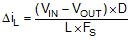Equation 14.D is the duty cycle of the converter which in a buck converter it can be approximated as D = VOUT / VIN, assuming no loss power conversion. By calculating in terms of amperes, volts, and megahertz, the inductance value will come out in micro Henries. The inductor ripple current ratio is defined by:

Equation 15.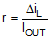The second criterion is the inductor saturation current rating. The inductor should be rated to handle the maximum load current plus the ripple current:

Equation 16. IL-PEAK = ILOAD-MAX + Δ iL

The LM43600-Q1 has both valley current limit and peak current limit. During an instantaneous short, the peak inductor current can be high due to a momentary increase in duty cycle. The inductor current rating should be higher than the HS current limit. It is advised to select an inductor with a larger core saturation margin and preferably a softer roll off of the inductance value over load current.

In general, it is preferable to choose lower inductance in switching power supplies, because it usually corresponds to faster transient response, smaller DCR, and reduced size for more compact designs. But too low of an inductance can generate too large of an inductor current ripple such that over current protection at the full load could be falsely triggered. It also generates more conduction loss, since the RMS current is slightly higher relative that with lower current ripple at the same DC current. Larger inductor current ripple also implies larger output voltage ripple with the same output capacitors. With peak current mode control, it is not recommended to have too small of an inductor current ripple. Enough inductor current ripple improves signal-to-noise ratio on the current comparator and makes the control loop more immune to noise.

Once the inductance is determined, the type of inductor must be selected. Ferrite designs have very low core losses and are preferred at high switching frequencies, so design goals can concentrate on copper loss and preventing saturation. Ferrite core material saturates hard, which means that inductance collapses abruptly when the peak design current is exceeded. The ‘hard’ saturation results in an abrupt increase in inductor ripple current and consequent output voltage ripple. Do not allow the core to saturate!

For the design example, a standard 22 μH inductor from Würth, Coiltronics, or Vishay can be used for the 3.3 V output with plenty of current rating margin.

#### 8.2.2.6 Output Capacitor Selection

The device is designed to be used with a wide variety of LC filters. It is generally desired to use as little output capacitance as possible to keep cost and size down. The output capacitor (s), COUT, should be chosen with care since it directly affects the steady state output voltage ripple, loop stability and the voltage over/undershoot during load current transients.

The output voltage ripple is essentially composed of two parts. One is caused by the inductor current ripple going through the equivalent series resistance (ESR) of the output capacitors:

Equation 17. ΔVOUT-ESR = ΔiL × ESR

The other is caused by the inductor current ripple charging and discharging the output capacitors:

Equation 18. ΔVOUT-C = ΔiL/ ( 8 × FS × COUT )

The two components in the voltage ripple are not in phase, so the actual peak-to-peak ripple is smaller than the sum of the two peaks.

Output capacitance is usually limited by transient performance specifications if the system requires tight voltage regulation in the presence of large current steps and fast slew rates. When a fast large load transient happens, output capacitors provide the required charge before the inductor current can slew to the appropriate level. The initial output voltage step is equal to the load current step multiplied by the ESR. VOUT continues to droop until the control loop response increases or decreases the inductor current to supply the load. To maintain a small over- or under-shoot during a transient, small ESR and large capacitance are desired. But these also come with higher cost and size. Thus, the motivation is to seek a fast control loop response to reduce the output voltage deviation.

For a given input and output requirement, Equation 19 an approximation for an absolute minimum output capacitr required:

Equation 19.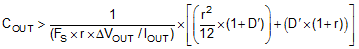Along with this for the same requirement, calculate the maximum ESR per Equation 20:

Equation 20.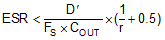where

• r = Ripple ratio of the inductor ripple current (ΔIL / IOUT)
• ΔVOUT = target output voltage undershoot
• D’ = 1 – duty cycle
• FS = switching frequency

A general guideline for COUT range is that COUT should be larger than the minimum required output capacitance calculated by Equation 19, and smaller than 10 times the minimum required output capacitance or 1 mF. In applications with VOUT less than 3.3 V, it is critical that low ESR output capacitors are selected. This will limit potential output voltage overshoots as the input voltage falls below the device normal operating range. To optimize the transient behavior a feed-forward capacitor could be added in parallel with the upper feedback resistor. For this design example, two 47 µF,10 V, X7R ceramic capacitors are used in parallel.

#### 8.2.2.7 Feedvorward Capacitor

The LM43600-Q1 is internally compensated and the internal R-C values are 400 kΩ and 50 pF, respectively. Depending on the VOUT and frequency FS, if the output capacitor COUT is dominated by low ESR (ceramic types) capacitors, it could result in low phase margin. To improve the phase boost an external feedforward capacitor CFF can be added in parallel with RFBT. CFF is chosen such that phase margin is boosted at the crossover frequency without CFF. A simple estimation for the crossover frequency without CFF (fx) is shown in Equation 21, assuming COUT has very small ESR.

Equation 21.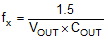Equation 22 for CFF was tested:

Equation 22.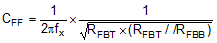Equation 22 indicates that the crossover frequency is geometrically centered on the zero and pole frequencies caused by the CFF capacitor.

For designs with higher ESR, CFF is not needed when COUT has very high ESR and CFF calculated from Equation 22 should be reduced with medium ESR. Table 2 can be used as a quick starting point.

For the application in this design example, a 33-pF COG capacitor is selected.

#### 8.2.2.8 Bootstrap Capacitors

Every LM43600-Q1 design requires a bootstrap capacitor, CBOOT. The recommended bootstrap capacitor is 0.47 μF and rated at 6.3 V or higher. The bootstrap capacitor is located between the SW pin and the CBOOT pin. The bootstrap capacitor must be a high-quality ceramic type with X7R or X5R grade dielectric for temperature stability.

#### 8.2.2.9 VCC Capacitor

The VCC pin is the output of an internal LDO for LM43600-Q1. The input for this LDO comes from either VIN or BIAS (please refer to Functional Block Diagram for LM43600-Q1). To insure stability of the part, place a minimum of 2.2 µF, 10 V capacitor from this pin to ground.

#### 8.2.2.10 BIAS Capacitors

For an output voltage of 3.3 V and greater, the BIAS pin can be connected to the output in order to increase light load efficiency. This pin is an input for the VCC LDO. When BIAS is not connected, the input for the VCC LDO will be internally connected into VIN. Because this is an LDO, the voltage differences between the input and output will affect the efficiency of the LDO. If necessary, a capacitor with a value of 1 μF can be added close to the BIAS pin as an input capacitor for the LDO.

#### 8.2.2.11 Soft-Start Capacitors

The user can leave the SS/TRK pin floating, and the LM43600-Q1 will implement a soft start time of 4.1 ms typically. In order to use an external soft start capacitor, the capacitor should be sized such that the soft start time will be longer than 4.1 ms. Use the following equation in order to calculate the soft start capacitor value:

Equation 23.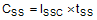where

• CSS = soft-start capacitor value (µF)
• ISS = soft-start charging current (µA)
• tSS = desired soft-start time (s)

For the desired soft-start time of 10 ms and soft start charging current of 2.2 µA, Equation 23 yields a soft-start capacitor value of 0.022 µF.

#### 8.2.2.12 Undervoltage Lockout Setpoint

The undervoltage lockout (UVLO) is adjusted using the external voltage divider network of RENT and RENB. RENT is connected between VIN and the EN pin of the LM43600-Q1 device. RENB is connected between the EN pin and the GND pin. The UVLO has two thresholds, one for power up when the input voltage is rising and one for power down or brown outs when the input voltage is falling. The following equation can be used to determine the VIN (UVLO) level.

Equation 24. VEN = VIN-UVLO × RENB / (RENB + RENT)

The EN rising threshold for LM43600-Q1 is set to be 2.2 V. Choose the value of RENB to be 1 MΩ to minimize input current going into the converter. If the desired VIN (UVLO) level is at 3.5 V, then the value of RENT can be calculated using the equation above and yield a value of 590 kΩ.

#### 8.2.2.13 PGOOD

A typical pullup resistor value is 10 kΩ to 100 kΩ from the PGOOD pin to a voltage no higher than 12 V. If it is desired to pull up the PGOOD pin to a voltage higher than 12 V, a resistor can be added from the PGOOD pin to ground to divide the voltage seen by the PGOOD pin to a value no higher than 12 V.

#### 8.2.3 Application Performance Curves

See Table 2 for bill of materials for each VOUT and FS combination. Unless otherwise stated, application performance curves were taken at TA = 25 °C.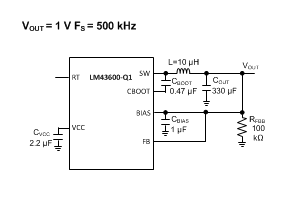VOUT = 1 V FS = 500 kHz VIN = 12 V
Figure 47. BOM for VOUT = 1 V FS = 500 kHz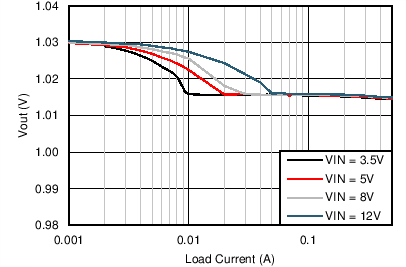VOUT = 1 V FS = 500 kHz
Figure 49. Output Voltage Regulation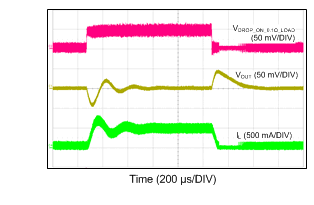VOUT = 1 V FS = 500 kHz VIN = 12 V
Figure 51. Load Transient Between 0.05 A and 0.5 A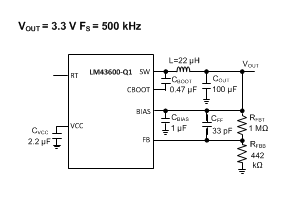VOUT = 3.3 V FS = 500 kHz VIN = 12 V
Figure 53. BOM for VOUT = 3.3 V FS = 500 kHz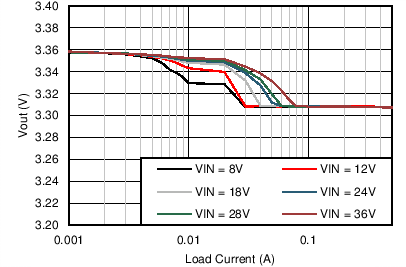VOUT = 3.3 V FS = 500 kHz
Figure 55. Output Voltage Regulation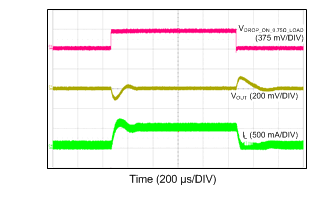VOUT = 3.3 V FS = 500 kHz VIN = 12 V
Figure 57. Load Transient Between 0.05 A and 0.5 A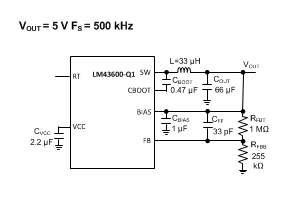VOUT = 5 V FS = 500 kHz VIN = 12 V
Figure 59. BOM for VOUT = 5 V FS = 500 kHz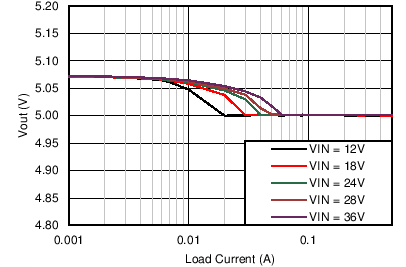VOUT = 5 V FS = 500 kHz
Figure 61. Output Voltage Regulation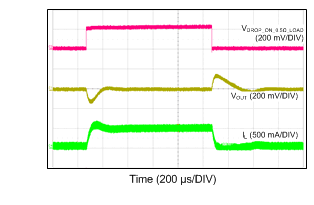VOUT = 5 V FS = 500 kHz VIN = 12 V
Figure 63. Load Transient Between 0.05 A and 0.5 A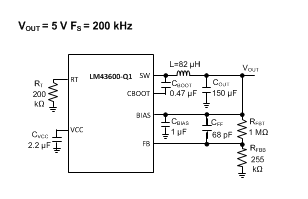VOUT = 5 V FS = 200 kHz VIN = 12 V
Figure 65. BOM for VOUT = 5 V FS = 200 kHz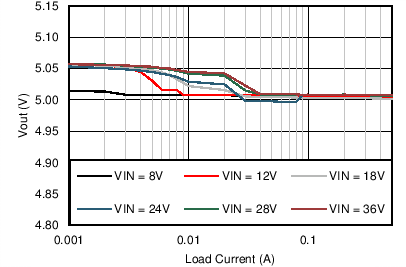VOUT = 5 V FS = 200 kHz
Figure 67. Output Voltage RegulationVOUT = 5 V FS = 200 kHz VIN = 12 V
Figure 69. Load Transient Between 0.05 A and 0.5 A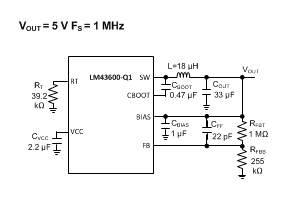VOUT = 5 V FS = 1 MHz VIN = 12 V
Figure 71. BOM for VOUT = 5 V FS = 1 MHz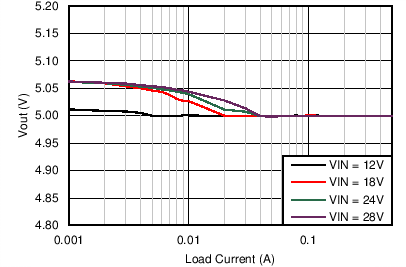VOUT = 5 V FS = 1 MHz
Figure 73. Output Voltage Regulation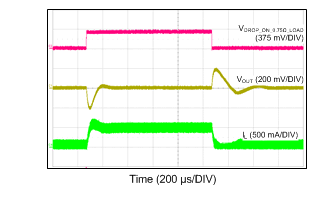VOUT = 5 V FS = 1 MHz VIN = 12 V
Figure 75. Load Transient Between 0.05 A and 0.5 A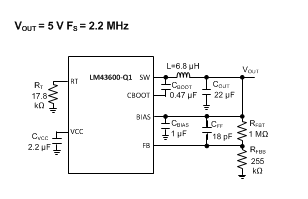VOUT = 5 V FS = 2.2 MHz VIN = 12 V
Figure 77. BOM for VOUT = 5 V FS = 2.2 MHz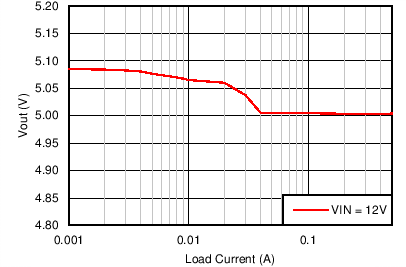VOUT = 5 V FS = 2.2 MHz
Figure 79. Output Voltage Regulation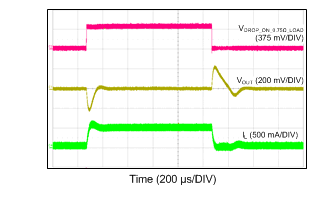VOUT = 5 V FS = 2.2 MHz VIN = 12 V
Figure 81. Load Transient Between 0.05 A and 0.5 A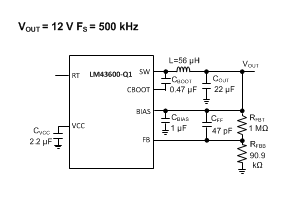VOUT = 12 V FS = 500 kHz VIN = 24 V
Figure 83. BOM for VOUT = 12 V FS = 500 kHz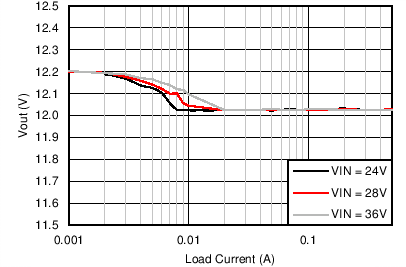VOUT = 12 V FS = 500 kHz
Figure 85. Output Voltage Regulation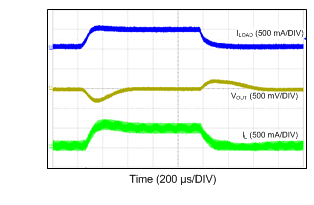VOUT = 12 V FS = 500 kHz VIN = 24 V
Figure 87. Load Transient Between 0.05 A and 0.5 A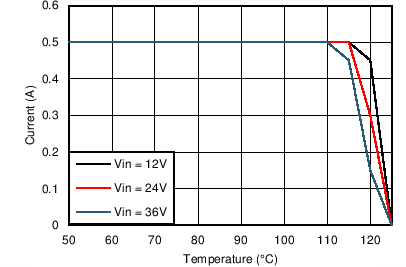VOUT = 3.3 V FS = 500 kHz RθJA = 20 °C/W
Figure 89. Derating Curve with RθJA = 20 °C/W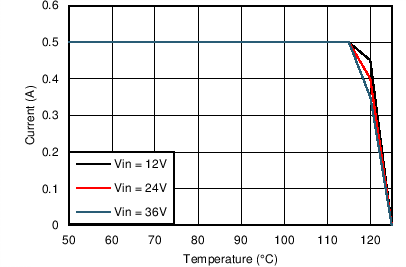VOUT = 5 V FS = 200 kHz RθJA = 20 °C/W
Figure 91. Derating Curve with RθJA = 20 °C/W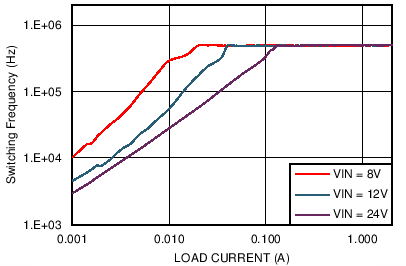VOUT = 3.3 V FS = 500 kHz
Figure 93. Switching Frequency vs IOUT in PFM Operation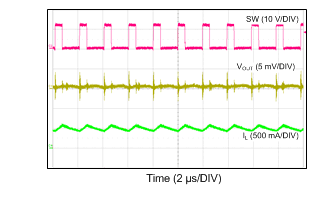VOUT = 3.3 V FS = 500 kHz IOUT = 0.5 A
Figure 95. Switching Waveform in CCM Operation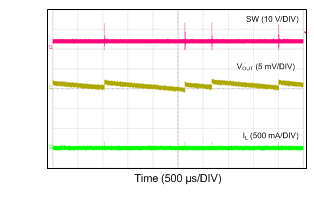VOUT = 3.3 V FS = 500 kHz IOUT = 0 mA
Figure 97. Switching Waveform in PFM Operation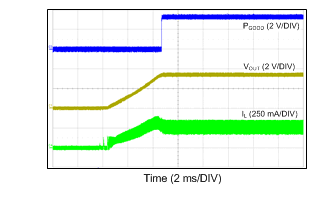VIN = 12 V VOUT = 3.3 V RLOAD = 13.2 Ω
Figure 99. Startup Into Half Load with Internal Soft-Start Rate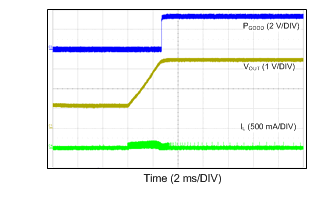VIN = 12 V VOUT = 3.3 V RLOAD = Open
Figure 101. Startup Into 1 V Pre-biased Voltage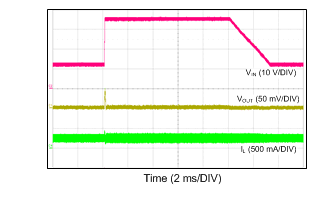VOUT = 3.3 V FS = 500 kHz IOUT = 0.25 A
Figure 103. Line Transient: VIN Transitions Between 12 V and 36 V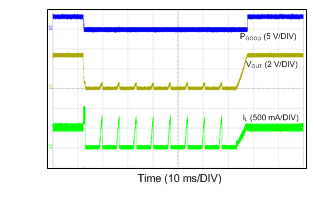VOUT = 3.3 V FS = 500 kHz VIN = 12 V
Figure 105. Short-Circuit Protection and Recover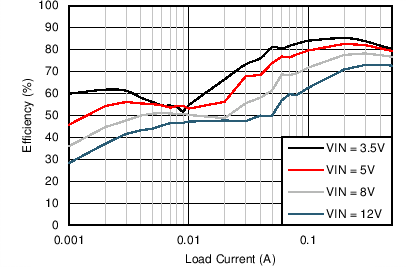VOUT = 1 V FS = 500 kHz
Figure 48. Efficiency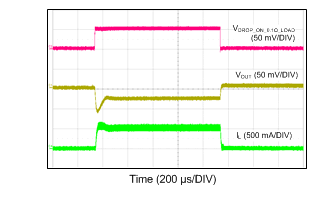VOUT = 1 V FS = 500 kHz VIN = 12 V
Figure 50. Load Transient Between 0 A and 0.5 A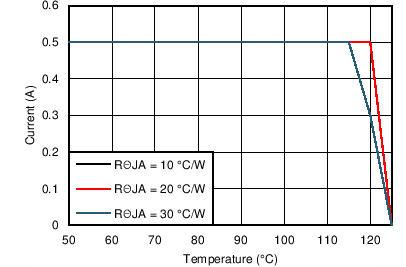VOUT = 1 V FS = 500 kHz VIN = 12 V
Figure 52. Derating Curve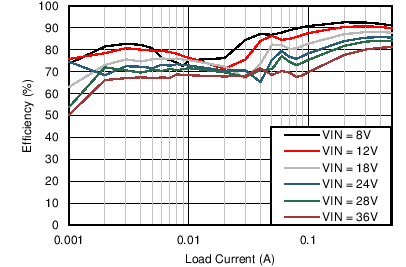VOUT = 3.3 V FS = 500 kHz
Figure 54. Efficiency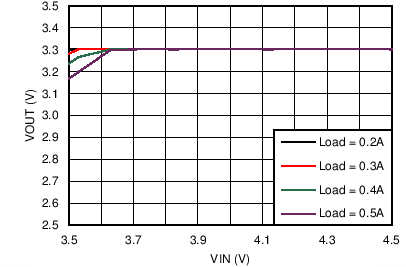VOUT = 3.3 V FS = 500 kHz
Figure 56. Dropout Curve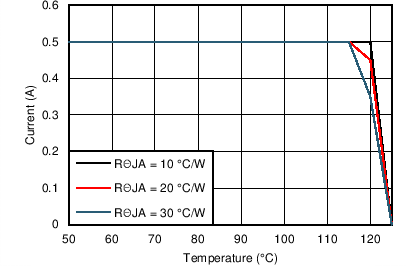VOUT = 3.3 V FS = 500 kHz VIN = 12 V
Figure 58. Derating Curve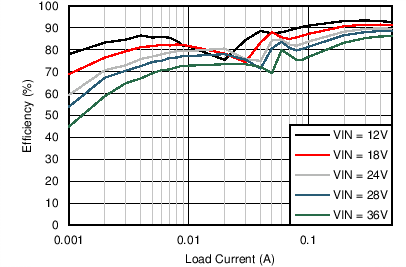VOUT = 5 V FS = 500 kHz
Figure 60. Efficiency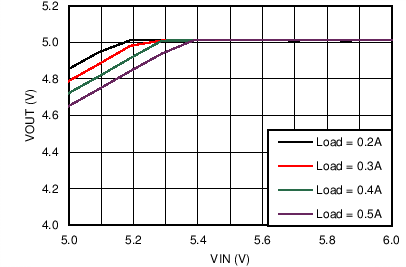VOUT = 5 V FS = 500 kHz
Figure 62. Dropout Curve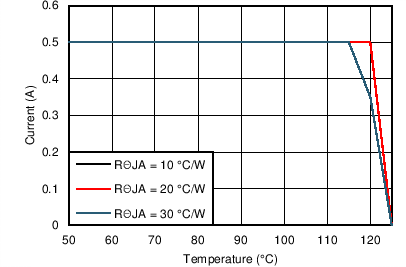VOUT = 5 V FS = 500 kHz VIN = 12 V
Figure 64. Derating Curve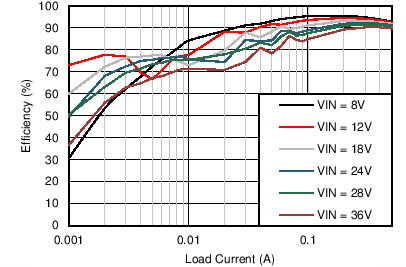VOUT = 5 V FS = 200 kHz
Figure 66. EfficiencyVOUT = 5 V FS = 200 kHz
Figure 68. Dropout CurveVOUT = 5 V FS = 200 kHz VIN = 12 V
Figure 70. Derating Curve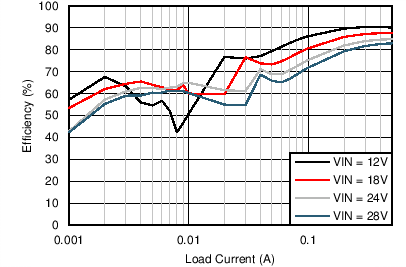VOUT = 5 V FS = 1 MHz VIN = 12 V
Figure 72. Efficiency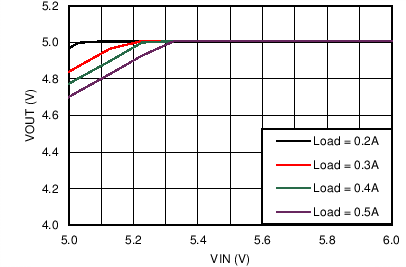VOUT = 5 V FS = 1 MHz
Figure 74. Dropout Curve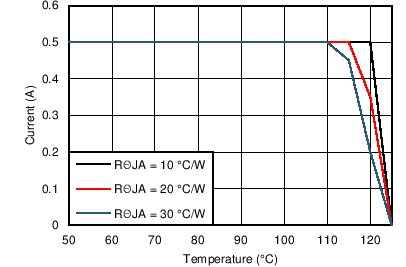VOUT = 5 V FS = 1 MHz VIN = 12 V
Figure 76. Derating Curve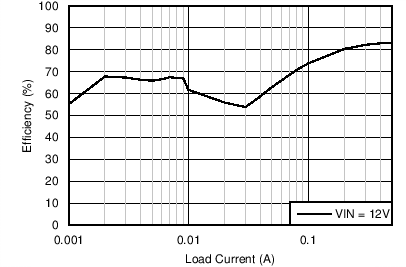VOUT = 5 V FS = 2.2 MHz VIN = 12 V
Figure 78. Efficiency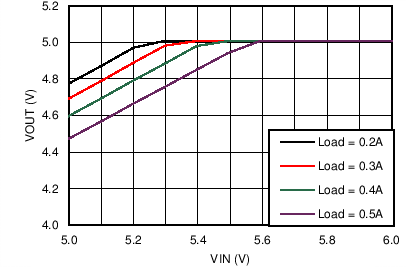VOUT = 5 V FS = 2.2 MHz
Figure 80. Dropout Curve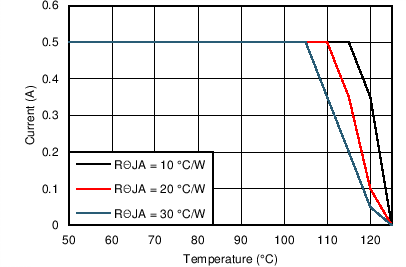VOUT = 5 V FS = 2.2 MHz VIN = 12 V
Figure 82. Derating CurveVOUT = 12 V FS = 500 kHz
Figure 84. Efficiency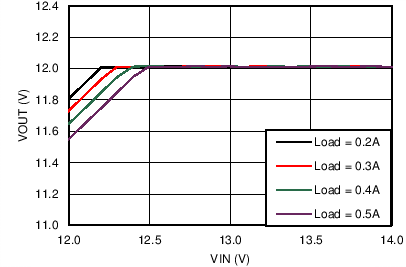VOUT = 12 V FS = 500 kHz
Figure 86. Dropout Curve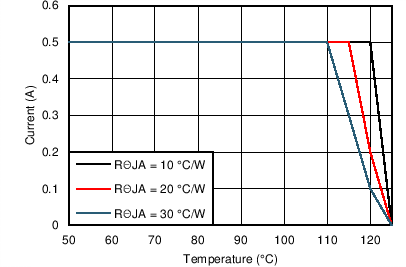VOUT = 12 V FS = 500 kHz VIN = 24 V
Figure 88. Derating Curve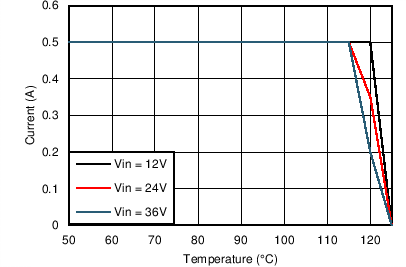VOUT = 5 V FS = 500 kHz RθJA = 20 °C/W
Figure 90. Derating Curve with RθJA = 20 °C/W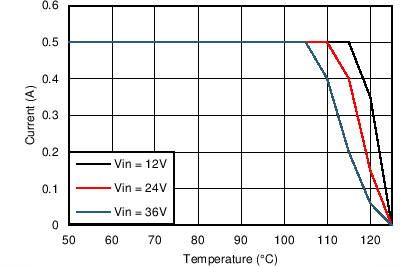VOUT = 5 V FS = 1 MHz RθJA = 20 °C/W
Figure 92. Derating Curve with RθJA = 20 °C/W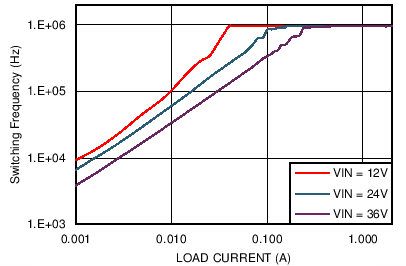VOUT = 5 V FS = 1 MHz
Figure 94. Switching Frequency vs IOUT in PFM Operation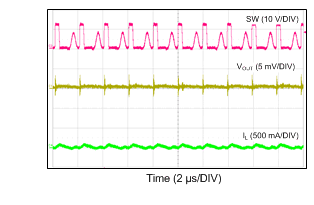VOUT = 3.3 V FS = 500 kHz IOUT =10 mA
Figure 96. Switching Waveform in DCM Operation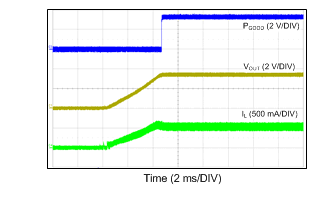VIN = 12 V VOUT = 3.3 V RLOAD = 6.6 Ω
Figure 98. Startup Into Full Load with Internal Soft-Start Rate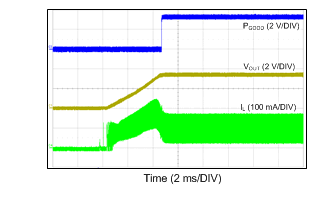VIN = 12 V VOUT = 3.3 V RLOAD = 33 Ω
Figure 100. Startup Into 100 mA with Internal Soft-Start Rate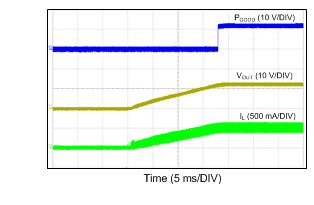VIN = 24 V VOUT = 12 V RLOAD = 6 Ω
Figure 102. Startup with External Capacitor CSS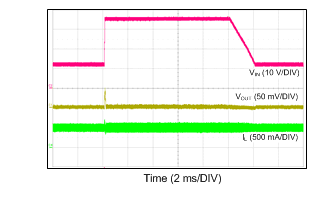VOUT = 3.3 V FS = 500 kHz IOUT = 0.5 A
Figure 104. Line Transient: VIN Transitions Between 12 V and 36 V